Publicité

# Vlsi lab viva question with answers

5 Jan 2013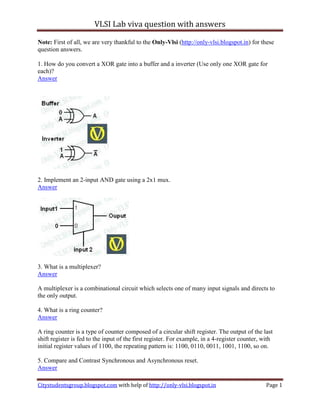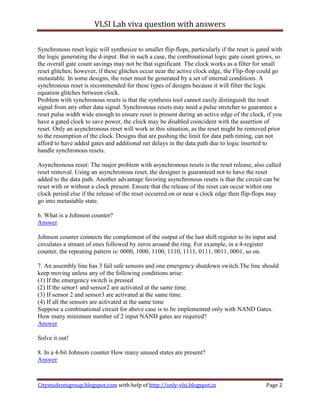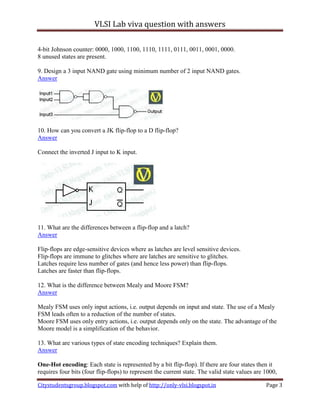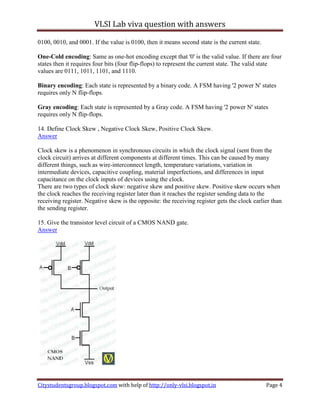Publicité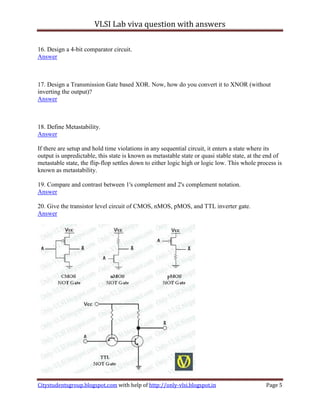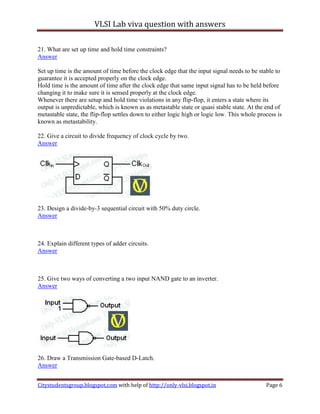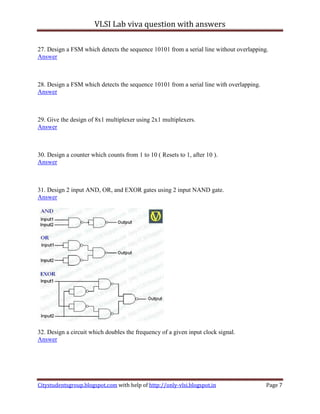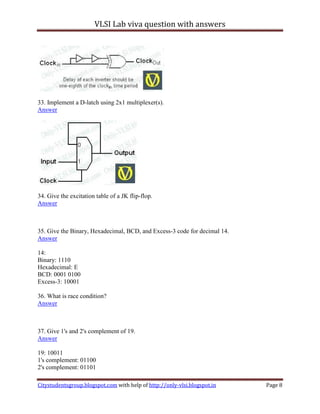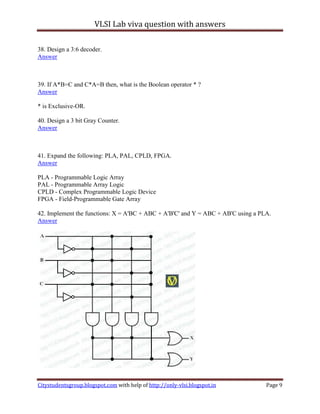Publicité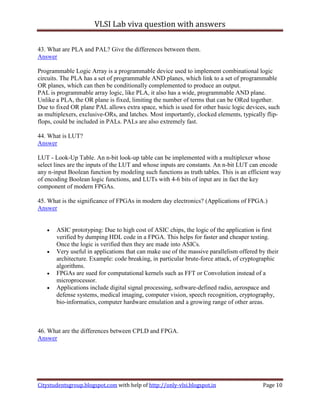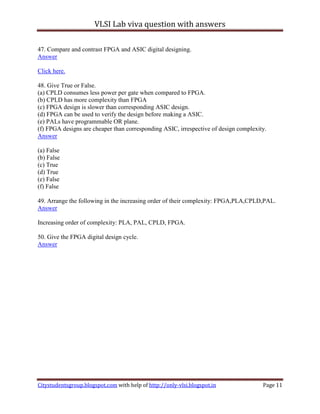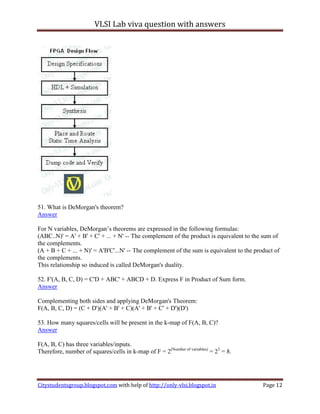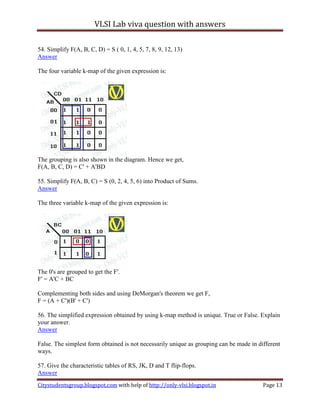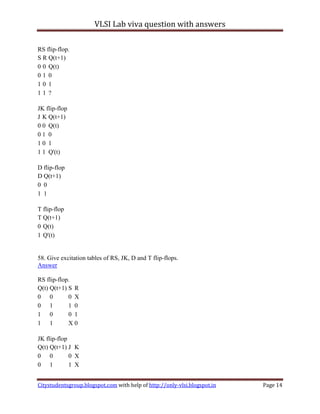Publicité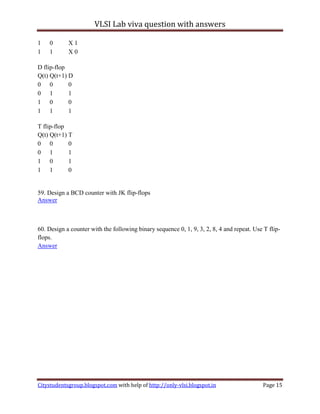Prochain SlideShare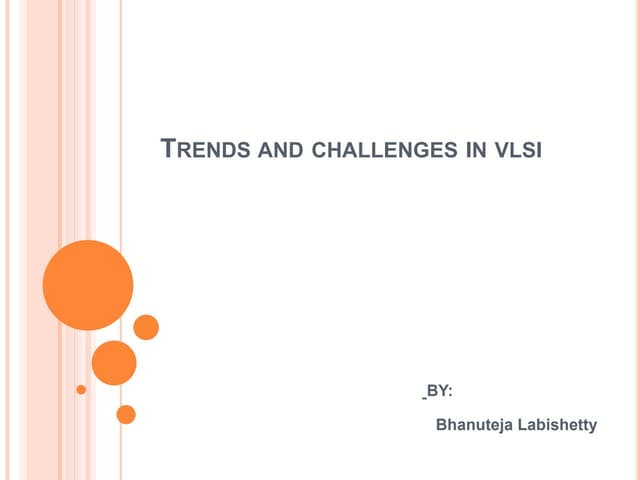Trends and challenges in vlsi
Chargement dans ... 3
1 sur 15
Publicité

### Vlsi lab viva question with answers

1. VLSI Lab viva question with answers Note: First of all, we are very thankful to the Only-Vlsi (http://only-vlsi.blogspot.in) for these question answers. 1. How do you convert a XOR gate into a buffer and a inverter (Use only one XOR gate for each)? Answer 2. Implement an 2-input AND gate using a 2x1 mux. Answer 3. What is a multiplexer? Answer A multiplexer is a combinational circuit which selects one of many input signals and directs to the only output. 4. What is a ring counter? Answer A ring counter is a type of counter composed of a circular shift register. The output of the last shift register is fed to the input of the first register. For example, in a 4-register counter, with initial register values of 1100, the repeating pattern is: 1100, 0110, 0011, 1001, 1100, so on. 5. Compare and Contrast Synchronous and Asynchronous reset. Answer Citystudentsgroup.blogspot.com with help of http://only-vlsi.blogspot.in Page 1
2. VLSI Lab viva question with answers Synchronous reset logic will synthesize to smaller flip-flops, particularly if the reset is gated with the logic generating the d-input. But in such a case, the combinational logic gate count grows, so the overall gate count savings may not be that significant. The clock works as a filter for small reset glitches; however, if these glitches occur near the active clock edge, the Flip-flop could go metastable. In some designs, the reset must be generated by a set of internal conditions. A synchronous reset is recommended for these types of designs because it will filter the logic equation glitches between clock. Problem with synchronous resets is that the synthesis tool cannot easily distinguish the reset signal from any other data signal. Synchronous resets may need a pulse stretcher to guarantee a reset pulse width wide enough to ensure reset is present during an active edge of the clock, if you have a gated clock to save power, the clock may be disabled coincident with the assertion of reset. Only an asynchronous reset will work in this situation, as the reset might be removed prior to the resumption of the clock. Designs that are pushing the limit for data path timing, can not afford to have added gates and additional net delays in the data path due to logic inserted to handle synchronous resets. Asynchronous reset: The major problem with asynchronous resets is the reset release, also called reset removal. Using an asynchronous reset, the designer is guaranteed not to have the reset added to the data path. Another advantage favoring asynchronous resets is that the circuit can be reset with or without a clock present. Ensure that the release of the reset can occur within one clock period else if the release of the reset occurred on or near a clock edge then flip-flops may go into metastable state. 6. What is a Johnson counter? Answer Johnson counter connects the complement of the output of the last shift register to its input and circulates a stream of ones followed by zeros around the ring. For example, in a 4-register counter, the repeating pattern is: 0000, 1000, 1100, 1110, 1111, 0111, 0011, 0001, so on. 7. An assembly line has 3 fail safe sensors and one emergency shutdown switch.The line should keep moving unless any of the following conditions arise: (1) If the emergency switch is pressed (2) If the senor1 and sensor2 are activated at the same time. (3) If sensor 2 and sensor3 are activated at the same time. (4) If all the sensors are activated at the same time Suppose a combinational circuit for above case is to be implemented only with NAND Gates. How many minimum number of 2 input NAND gates are required? Answer Solve it out! 8. In a 4-bit Johnson counter How many unused states are present? Answer Citystudentsgroup.blogspot.com with help of http://only-vlsi.blogspot.in Page 2
3. VLSI Lab viva question with answers 4-bit Johnson counter: 0000, 1000, 1100, 1110, 1111, 0111, 0011, 0001, 0000. 8 unused states are present. 9. Design a 3 input NAND gate using minimum number of 2 input NAND gates. Answer 10. How can you convert a JK flip-flop to a D flip-flop? Answer Connect the inverted J input to K input. 11. What are the differences between a flip-flop and a latch? Answer Flip-flops are edge-sensitive devices where as latches are level sensitive devices. Flip-flops are immune to glitches where are latches are sensitive to glitches. Latches require less number of gates (and hence less power) than flip-flops. Latches are faster than flip-flops. 12. What is the difference between Mealy and Moore FSM? Answer Mealy FSM uses only input actions, i.e. output depends on input and state. The use of a Mealy FSM leads often to a reduction of the number of states. Moore FSM uses only entry actions, i.e. output depends only on the state. The advantage of the Moore model is a simplification of the behavior. 13. What are various types of state encoding techniques? Explain them. Answer One-Hot encoding: Each state is represented by a bit flip-flop). If there are four states then it requires four bits (four flip-flops) to represent the current state. The valid state values are 1000, Citystudentsgroup.blogspot.com with help of http://only-vlsi.blogspot.in Page 3
4. VLSI Lab viva question with answers 0100, 0010, and 0001. If the value is 0100, then it means second state is the current state. One-Cold encoding: Same as one-hot encoding except that '0' is the valid value. If there are four states then it requires four bits (four flip-flops) to represent the current state. The valid state values are 0111, 1011, 1101, and 1110. Binary encoding: Each state is represented by a binary code. A FSM having '2 power N' states requires only N flip-flops. Gray encoding: Each state is represented by a Gray code. A FSM having '2 power N' states requires only N flip-flops. 14. Define Clock Skew , Negative Clock Skew, Positive Clock Skew. Answer Clock skew is a phenomenon in synchronous circuits in which the clock signal (sent from the clock circuit) arrives at different components at different times. This can be caused by many different things, such as wire-interconnect length, temperature variations, variation in intermediate devices, capacitive coupling, material imperfections, and differences in input capacitance on the clock inputs of devices using the clock. There are two types of clock skew: negative skew and positive skew. Positive skew occurs when the clock reaches the receiving register later than it reaches the register sending data to the receiving register. Negative skew is the opposite: the receiving register gets the clock earlier than the sending register. 15. Give the transistor level circuit of a CMOS NAND gate. Answer Citystudentsgroup.blogspot.com with help of http://only-vlsi.blogspot.in Page 4
5. VLSI Lab viva question with answers 16. Design a 4-bit comparator circuit. Answer 17. Design a Transmission Gate based XOR. Now, how do you convert it to XNOR (without inverting the output)? Answer 18. Define Metastability. Answer If there are setup and hold time violations in any sequential circuit, it enters a state where its output is unpredictable, this state is known as metastable state or quasi stable state, at the end of metastable state, the flip-flop settles down to either logic high or logic low. This whole process is known as metastability. 19. Compare and contrast between 1's complement and 2's complement notation. Answer 20. Give the transistor level circuit of CMOS, nMOS, pMOS, and TTL inverter gate. Answer Citystudentsgroup.blogspot.com with help of http://only-vlsi.blogspot.in Page 5
6. VLSI Lab viva question with answers 21. What are set up time and hold time constraints? Answer Set up time is the amount of time before the clock edge that the input signal needs to be stable to guarantee it is accepted properly on the clock edge. Hold time is the amount of time after the clock edge that same input signal has to be held before changing it to make sure it is sensed properly at the clock edge. Whenever there are setup and hold time violations in any flip-flop, it enters a state where its output is unpredictable, which is known as as metastable state or quasi stable state. At the end of metastable state, the flip-flop settles down to either logic high or logic low. This whole process is known as metastability. 22. Give a circuit to divide frequency of clock cycle by two. Answer 23. Design a divide-by-3 sequential circuit with 50% duty circle. Answer 24. Explain different types of adder circuits. Answer 25. Give two ways of converting a two input NAND gate to an inverter. Answer 26. Draw a Transmission Gate-based D-Latch. Answer Citystudentsgroup.blogspot.com with help of http://only-vlsi.blogspot.in Page 6
7. VLSI Lab viva question with answers 27. Design a FSM which detects the sequence 10101 from a serial line without overlapping. Answer 28. Design a FSM which detects the sequence 10101 from a serial line with overlapping. Answer 29. Give the design of 8x1 multiplexer using 2x1 multiplexers. Answer 30. Design a counter which counts from 1 to 10 ( Resets to 1, after 10 ). Answer 31. Design 2 input AND, OR, and EXOR gates using 2 input NAND gate. Answer 32. Design a circuit which doubles the frequency of a given input clock signal. Answer Citystudentsgroup.blogspot.com with help of http://only-vlsi.blogspot.in Page 7
8. VLSI Lab viva question with answers 33. Implement a D-latch using 2x1 multiplexer(s). Answer 34. Give the excitation table of a JK flip-flop. Answer 35. Give the Binary, Hexadecimal, BCD, and Excess-3 code for decimal 14. Answer 14: Binary: 1110 Hexadecimal: E BCD: 0001 0100 Excess-3: 10001 36. What is race condition? Answer 37. Give 1's and 2's complement of 19. Answer 19: 10011 1's complement: 01100 2's complement: 01101 Citystudentsgroup.blogspot.com with help of http://only-vlsi.blogspot.in Page 8
9. VLSI Lab viva question with answers 38. Design a 3:6 decoder. Answer 39. If A*B=C and C*A=B then, what is the Boolean operator * ? Answer * is Exclusive-OR. 40. Design a 3 bit Gray Counter. Answer 41. Expand the following: PLA, PAL, CPLD, FPGA. Answer PLA - Programmable Logic Array PAL - Programmable Array Logic CPLD - Complex Programmable Logic Device FPGA - Field-Programmable Gate Array 42. Implement the functions: X = A'BC + ABC + A'B'C' and Y = ABC + AB'C using a PLA. Answer Citystudentsgroup.blogspot.com with help of http://only-vlsi.blogspot.in Page 9
10. VLSI Lab viva question with answers 43. What are PLA and PAL? Give the differences between them. Answer Programmable Logic Array is a programmable device used to implement combinational logic circuits. The PLA has a set of programmable AND planes, which link to a set of programmable OR planes, which can then be conditionally complemented to produce an output. PAL is programmable array logic, like PLA, it also has a wide, programmable AND plane. Unlike a PLA, the OR plane is fixed, limiting the number of terms that can be ORed together. Due to fixed OR plane PAL allows extra space, which is used for other basic logic devices, such as multiplexers, exclusive-ORs, and latches. Most importantly, clocked elements, typically flip- flops, could be included in PALs. PALs are also extremely fast. 44. What is LUT? Answer LUT - Look-Up Table. An n-bit look-up table can be implemented with a multiplexer whose select lines are the inputs of the LUT and whose inputs are constants. An n-bit LUT can encode any n-input Boolean function by modeling such functions as truth tables. This is an efficient way of encoding Boolean logic functions, and LUTs with 4-6 bits of input are in fact the key component of modern FPGAs. 45. What is the significance of FPGAs in modern day electronics? (Applications of FPGA.) Answer • ASIC prototyping: Due to high cost of ASIC chips, the logic of the application is first verified by dumping HDL code in a FPGA. This helps for faster and cheaper testing. Once the logic is verified then they are made into ASICs. • Very useful in applications that can make use of the massive parallelism offered by their architecture. Example: code breaking, in particular brute-force attack, of cryptographic algorithms. • FPGAs are sued for computational kernels such as FFT or Convolution instead of a microprocessor. • Applications include digital signal processing, software-defined radio, aerospace and defense systems, medical imaging, computer vision, speech recognition, cryptography, bio-informatics, computer hardware emulation and a growing range of other areas. 46. What are the differences between CPLD and FPGA. Answer Citystudentsgroup.blogspot.com with help of http://only-vlsi.blogspot.in Page 10
11. VLSI Lab viva question with answers 47. Compare and contrast FPGA and ASIC digital designing. Answer Click here. 48. Give True or False. (a) CPLD consumes less power per gate when compared to FPGA. (b) CPLD has more complexity than FPGA (c) FPGA design is slower than corresponding ASIC design. (d) FPGA can be used to verify the design before making a ASIC. (e) PALs have programmable OR plane. (f) FPGA designs are cheaper than corresponding ASIC, irrespective of design complexity. Answer (a) False (b) False (c) True (d) True (e) False (f) False 49. Arrange the following in the increasing order of their complexity: FPGA,PLA,CPLD,PAL. Answer Increasing order of complexity: PLA, PAL, CPLD, FPGA. 50. Give the FPGA digital design cycle. Answer Citystudentsgroup.blogspot.com with help of http://only-vlsi.blogspot.in Page 11
12. VLSI Lab viva question with answers 51. What is DeMorgan's theorem? Answer For N variables, DeMorgan’s theorems are expressed in the following formulas: (ABC..N)' = A' + B' + C' + ... + N' -- The complement of the product is equivalent to the sum of the complements. (A + B + C + ... + N)' = A'B'C'...N' -- The complement of the sum is equivalent to the product of the complements. This relationship so induced is called DeMorgan's duality. 52. F'(A, B, C, D) = C'D + ABC' + ABCD + D. Express F in Product of Sum form. Answer Complementing both sides and applying DeMorgan's Theorem: F(A, B, C, D) = (C + D')(A' + B' + C)(A' + B' + C' + D')(D') 53. How many squares/cells will be present in the k-map of F(A, B, C)? Answer F(A, B, C) has three variables/inputs. Therefore, number of squares/cells in k-map of F = 2(Number of variables) = 23 = 8. Citystudentsgroup.blogspot.com with help of http://only-vlsi.blogspot.in Page 12
13. VLSI Lab viva question with answers 54. Simplify F(A, B, C, D) = S ( 0, 1, 4, 5, 7, 8, 9, 12, 13) Answer The four variable k-map of the given expression is: The grouping is also shown in the diagram. Hence we get, F(A, B, C, D) = C' + A'BD 55. Simplify F(A, B, C) = S (0, 2, 4, 5, 6) into Product of Sums. Answer The three variable k-map of the given expression is: The 0's are grouped to get the F'. F' = A'C + BC Complementing both sides and using DeMorgan's theorem we get F, F = (A + C')(B' + C') 56. The simplified expression obtained by using k-map method is unique. True or False. Explain your answer. Answer False. The simplest form obtained is not necessarily unique as grouping can be made in different ways. 57. Give the characteristic tables of RS, JK, D and T flip-flops. Answer Citystudentsgroup.blogspot.com with help of http://only-vlsi.blogspot.in Page 13
14. VLSI Lab viva question with answers RS flip-flop. S R Q(t+1) 0 0 Q(t) 01 0 10 1 11 ? JK flip-flop J K Q(t+1) 0 0 Q(t) 01 0 10 1 1 1 Q'(t) D flip-flop D Q(t+1) 0 0 1 1 T flip-flop T Q(t+1) 0 Q(t) 1 Q'(t) 58. Give excitation tables of RS, JK, D and T flip-flops. Answer RS flip-flop. Q(t) Q(t+1) S R 0 0 0 X 0 1 1 0 1 0 0 1 1 1 X0 JK flip-flop Q(t) Q(t+1) J K 0 0 0 X 0 1 1 X Citystudentsgroup.blogspot.com with help of http://only-vlsi.blogspot.in Page 14
15. VLSI Lab viva question with answers 1 0 X1 1 1 X0 D flip-flop Q(t) Q(t+1) D 0 0 0 0 1 1 1 0 0 1 1 1 T flip-flop Q(t) Q(t+1) T 0 0 0 0 1 1 1 0 1 1 1 0 59. Design a BCD counter with JK flip-flops Answer 60. Design a counter with the following binary sequence 0, 1, 9, 3, 2, 8, 4 and repeat. Use T flip- flops. Answer Citystudentsgroup.blogspot.com with help of http://only-vlsi.blogspot.in Page 15
Publicité